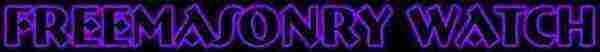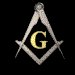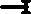Home# Grand Lodge of England Emulation Ritual Abbreviation Cipher CodesABBREVIATIONS

AND OTHER WORDS

•- gavel

• a d p o t t o t f j o t h - a distinct pressure of the thumb on the first joint of the hand

• a d p o t t b t f a s js o t h - a distinct pressure of the thumb between the first and second joints of the hand

• a d p o t t o t f s j o t h -

• a s p - a small pace

• Asst H P - Assistant High Priest

• b - bare

• b d t h s a t t a d i t t s -

• b i t s o t s at l w m - buried in the sands of the sea at low water mark

• C - Centre

• Cn -

• Cs - Compasses

• cs l f t s - cable's length from the sea

• Can - Candidate

• Cowan - Derogatory name for a non-mason

• c t - cable tow

• d...v...d - devoid

• d - distress

• d t l h, d t r s a t bt a d i t t s -

• E - East

• E A - Entered Apprentice

• E L of Imp -

• e...e - entrance

• e o c -

• f...t - feet

• F - Fidelity

• F C - Fellow Craft

• F M - Freemason

• f o w -

• F P O F - Five Points of Fellowship

• f r s - first real secret

• G and S O - Grand and Solemn oath

• G - Grip

• G A O T U - Great Architect of the Universe

• G G of D =

• G G U - Grand Geometrician of the Universe

• H A - Hiram Abif

• h - heart

• hs - heels

• h to h - heel to heel

• h i t p w t t e i t f o a s t t l o t w -

• h w - hoodwink

• h...w...d - hoodwinked

• I G - Inner Guard

• I P M - Immediate Past Master

• J D - Junior Deacon

• J W - Junior Warden

• k - knee

• k(s) - knock(s)

• K S - King Solomon

• K S T - King Solomon's Temple

• l a - left arm

• L - light

• Ls -

• l b - left breast

• l f - left foot

• l h p - left hand pillar

• l k - left knee

• M - Master

• m...c - metallic

• m...l - metal

• M M - Master Mason

• m...y - money

• Mn - Moon

• mys - mysteries

• n - neck

• N - North

• n l b - naked left breast

• Obl - obligation

• P and R in I -

• p -

• pts - point or points

• ped - pedestal

• p g - pass grip

• p...way - porchway

• P Sn - Pass sign

• pt on b - point on breast

• p t r h on t l b w t t e i t f o a s - putting the right hand on the left breast with the thumb extended in the form of a square

• r a - right arm or right angle

• r h - right hand

• r h p - right hand pillar

• r t -

• R Sn -

• S -

• Scts - secrets

• SD - Senior Deacon

• S of H -

• S of S -

• s i w y l t -

• Sn - Sign

• Sn of R -

• Sn of P -

• "So mote it

• S O - Solemn oath

• Sp -

• Sq - Square

• s r s -

• ss -

• s s -

• S W - Senior Warden

• t - token

• T - Tyler

• t c a - throat cut across

• Tn - Token

• t t o b t r - tongue torn out by the root

• t t r e a f t i 24hs - the tide ebbs and flows twice in 24 hours

• t u t l h w t t l e i t f o a s -

• v...l...e -

• V S L - Volume of Sacred Law

• W - West

• Wd - word

• W M - Worshipful Master

• w s -

• y t c a - your throat cut across